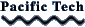### Learning Math with Graphing Calculator

If you have ordered Graphing Calculator, this book is already in the Graphing Calculator folder on your hard disk and you do not need to download it from here. It will be easier for you to read your local copy.

• Introduction
• What version do you have?
• How do you learn
• The Basics of Graphing Calculator
• Anatomy of the Graphing Calculator Window
• How do I zoom the graph in or out?
• How do I see other parts of the graph?
• How do I save my work?
• What if I need help?
• Using the Math Window
• How do I enter an equation?
• How do I select?
• What do I need to know about entering formulas
• Are there other ways to enter math stuff?
• How do I do fractions?
• How do I make exponents?
• Can I use Graphing Calculator as a calculator?
• Can I multiply out two parenthetical expressions?
• What about plain old arithmetic?
• How do I get more (or fewer) significant figures?
• How do I isolate a term?
• Can I do trig functions?
• Graphing
• How do I make a graph?
• How do I see different values for my parameters?
• Can I graph more than one function?
• Can I trace functions?
• Do I have to use x and y - and n - or can I use other letters?
• What should I know about constants and parameters?
• Graphing in Two Dimensions
• Polar coordinates
• Inequalities
• Transformations
• Translations, dilations, and y = mx + b
• Dilations: stretching, squishing, and flipping
• Putting transformations together
• Once more, with waves
• Choosing a parametrization
• Translations, the minus sign, and symmetry
• The difference between horizontal and vertical
• Exponentials
• Difference functions
• Fourier series
• Multiplying functions: envelopes
• Reciprocals of functions
• Inverse functions
• Functions of functions: composition
• Surfaces in 3D
• Basic 3D drawing in Graphing Calculator
• How to tell which axis is which
• Cool ways to slow down your computer
• A family of graphs at a glance
• Surfaces as genuine functions of two variables
• Surfaces of revolution
• Polar coordinates and surfaces
• Slicing 3D surfaces
• Conic sections - using cones!
• More general 3D surfaces
• Exploring Parametric Equations
• Remind me about parametric equations
• Making parametric curves in Graphing Calculator
• Lissajous fun
• Parametric equations without trig functions
• Making a spring: 3D parametric curves
• Parametric Surfaces
• Making the torus
• Changing the donut into a double helix
• Fancy Stuff
• Coloring
• Conditions
• If statements
• Summations
• Functions
• Symbolic calculus
• The difference between total and partial derivatives
• Ordinary differential equations
• Integrals
• Signum: a useful function to know about
• Vector fields
• But wait! There's more!
• Solving Problems with Graphing Calculator
• Word problems: the big picture
• What symbol manipulation is for
• Dimensional analysis
• Using substitution
• Figuring average speed